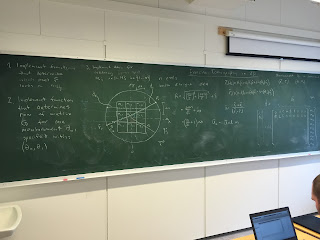### Inverse problems course

Some of our PhD students are busy taking a new "Practical inverse problems" course now. The course is held for the first time at UiT. The goal is to cover basic linear inverse problems, maximum a posterior estimates using SVD, statistical inverse problems, and non-linear problems. All of this in a practical setting, where all of the topics that are taught are also turned into programming exercises.

The first week was quite intense, starting with linear least squares, Bayesian statistics, and SVD. The second exercise was to solve the tomography problem. So far so good. Everyone taking the course seems to already have implemented their own tomographic reconstruction algorithm. Next up: different regularization methods, Fourier domain methods, robust norms,  non-linear inverse problems, linearized error analysis, and Markov chain Monte-Carlo sampling.Exercise 1 involves figuring out how to parametrize the tomography problem and how to implement it as a theory matrix.